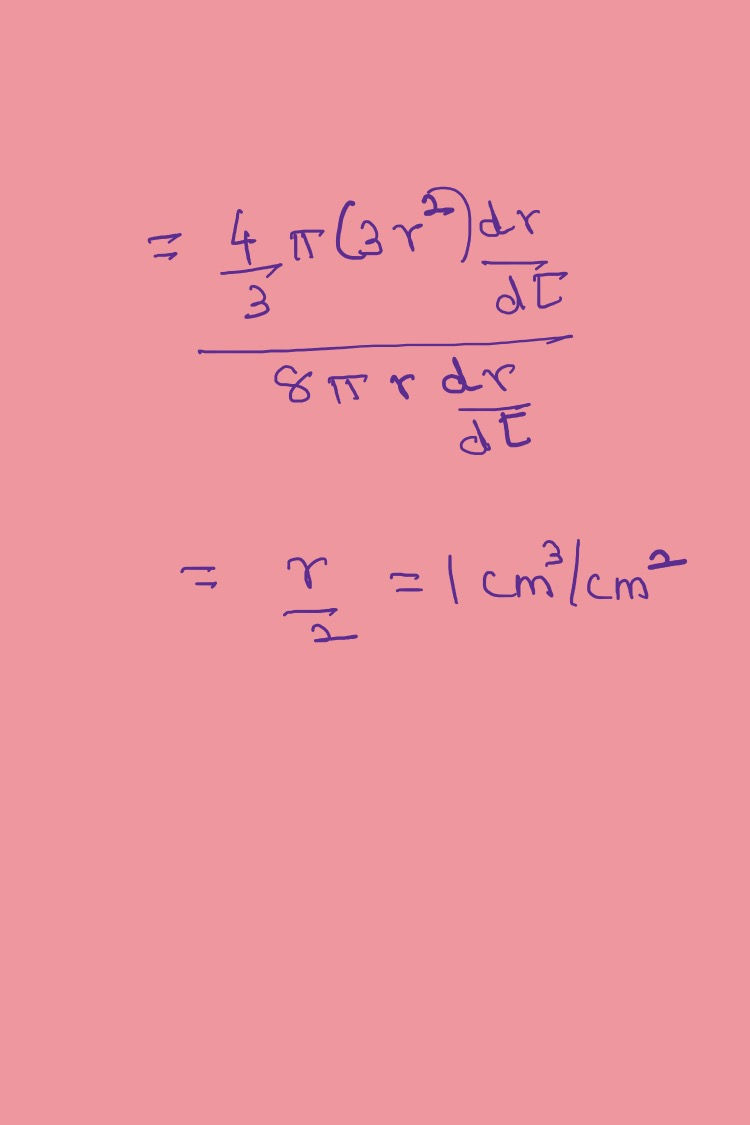top of page
Search

# How to Prepare for Applications of Derivatives-Class 12 Mathematics

Updated: Sep 10, 2022

## A ladder 5 m long rests against a vertical wall. If the top of the ladder slides downward at the rate of 10 cm/sec. find the rate at which the angle between the floor and the ladder is decreasing when the lower end of the ladder is 2 m away from the wall.## Find the coordinates of a point on the ellipse where the ordinate decreases at the same rate at which the abscissa increases.## Find the rate of change of volume of a sphere with respect to its surface area when the radius is 2 cm.rate of change questionrate of change solution slide 1## When there is a small change in x, there arises a small change in y, this is called the differential of y. The formula to calculate the Differential is illustrated here.